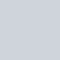# 範例程式碼

``````from functools import wraps
import time

def WongWongTimer(func):
@wraps(func)
def wrap(*args, **kargs):
time_start = time.time()
value = func(*args, **kargs)
time_end = time.time()
time_spend = time_end - time_start
#print(f"[{func.__module__}.{func.__name__}] cost time: {time_spend}")
print(f"[WongWongTimer][{func.__qualname__}] cost time: {time_spend}")

return value

return wrap
``````

## 使用方式 – 單純版，撰寫 wongwong_utils.py

• 範例如下：
``````from wongwong_utils import  WongWongTimer

@WongWongTimer
def test():
print("Hello World!!!")

test()
``````

## 使用方式 – 大型程式重複使用版 (放入指定資料夾並設定 `__init__.py`)

• main.py
• utils
• `__init__.py`
• `wongwong_utils.py`

`__init__`」.py 的功能，主要是讓一個資料夾內的所有內容可以被模組化，

### 撰寫 utils 資料夾底下的 init.py (使 utils 成為 package)

``````from .wongwong_utils import *
__all__ = ['WongWongTimer'']
``````

### 撰寫 utils 資料夾底下的 wongwong_utils.py

``````from functools import wraps
import time

def WongWongTimer(func):
@wraps(func)
def wrap(*args, **kargs):
time_start = time.time()
value = func(*args, **kargs)
time_end = time.time()
time_spend = time_end - time_start
#print(f"[{func.__module__}.{func.__name__}] cost time: {time_spend}")
print(f"[WongWongTimer][{func.__qualname__}] cost time: {time_spend}")

return value

return wrap
``````

### 實作範例

``````from utils import WongWongTimer

@WongWongTimer
def test():
print("Hello World!!!")

test()
``````

``````from utils import WongWongTimer
``````

(例如說我們想在 utils 資料夾底下這樣 import WongWongTimer，會找不到不能使用)

### 未使用 init.py 加工成 utils package 的方法也可以

from utils.wongwong_utils import WongWongTimer

# 延伸討論